# Slides & my Presentation style

this adds ?showNotes=true to the file

click this link for pdf printable + notes

HTML is best viewed in chrome

# What we want to be able to analyse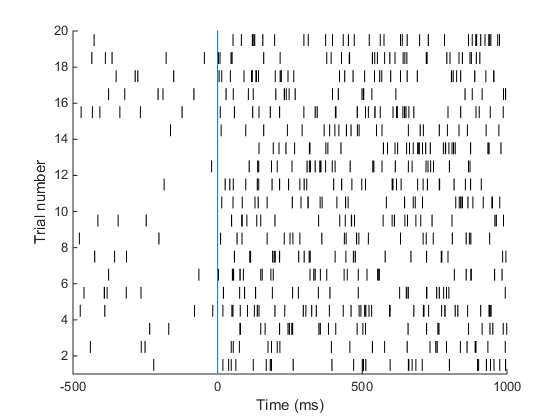Neural firing is poisson-like 1,2,3,4 .. spike / s => Discrete outcome!

GLMs allow us to model outcomes from distributions other than the normal distribution.

# Overview

• Binary Outcomes
• Example
• Logistic regression
• Units, odds, relative risks
• Interpretation of coefficients
• Checking the model
• Inference
• Poisson Regression
• GLMs
• Assumptions

## Example tasks with binomial data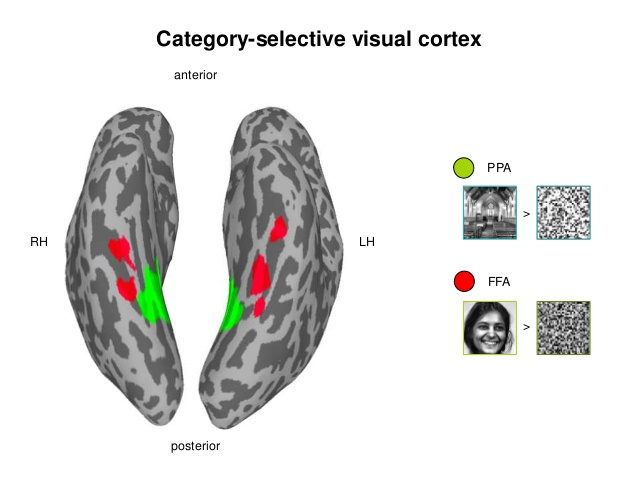Decoding: Is it a face or a house? Based on BOLD/EEG data

## Bernoulli vs Binomial

### Bernoulli

• A single throw of a coin
• Will the coin be heads or tails?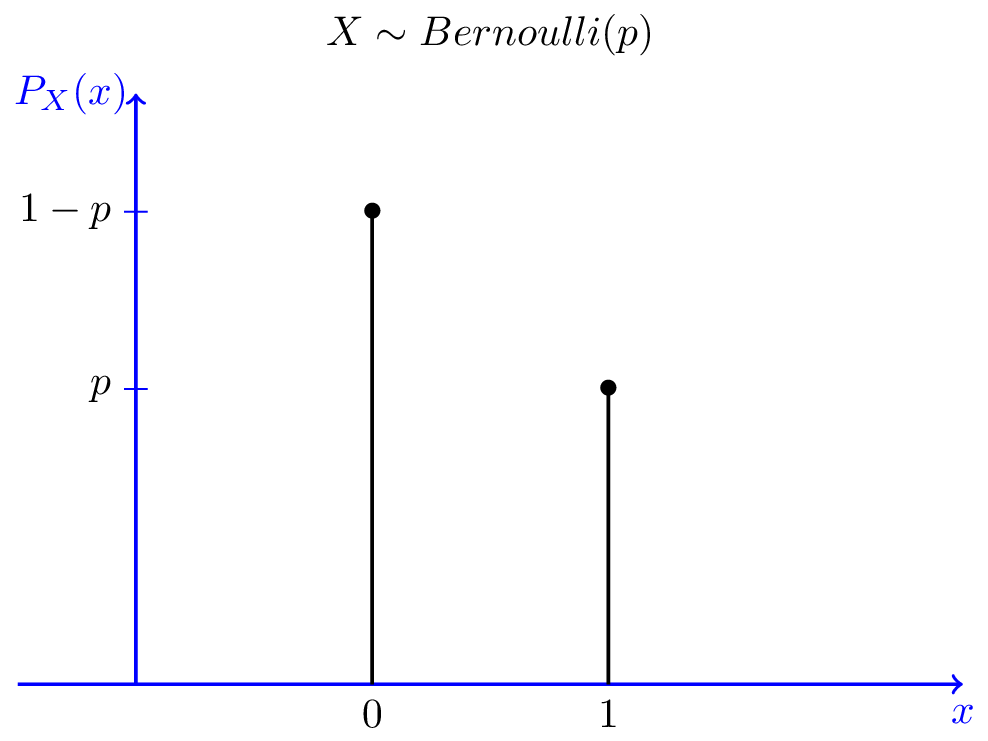## An example with a continuos variable

• Task: Do you observe a grating?
• Research Question: What is the minimum contrast to reliably (>25%) detect a grating?n.trial =150
n.contrastLevels=12

## The Data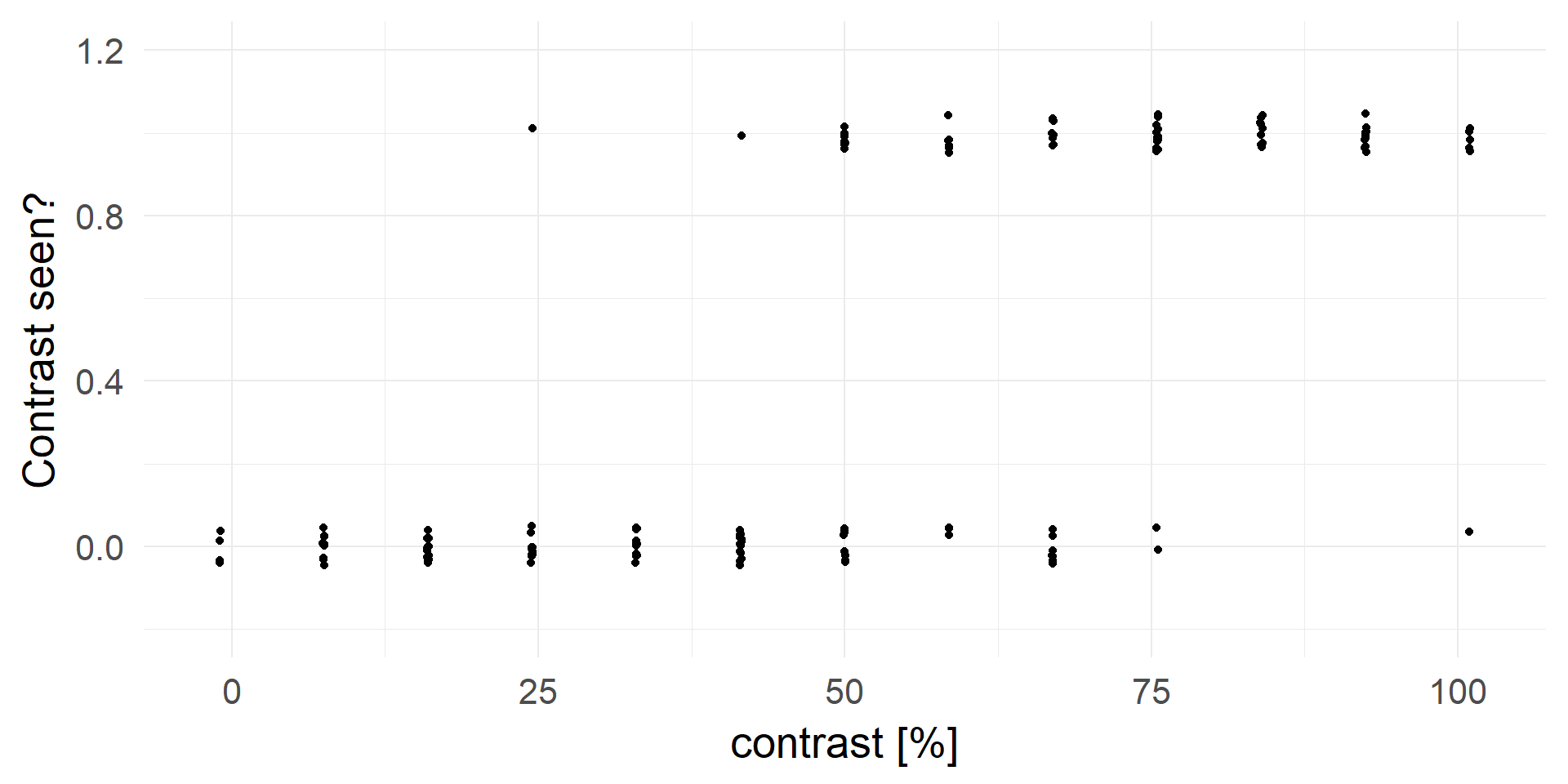## What is the probability to detect the target?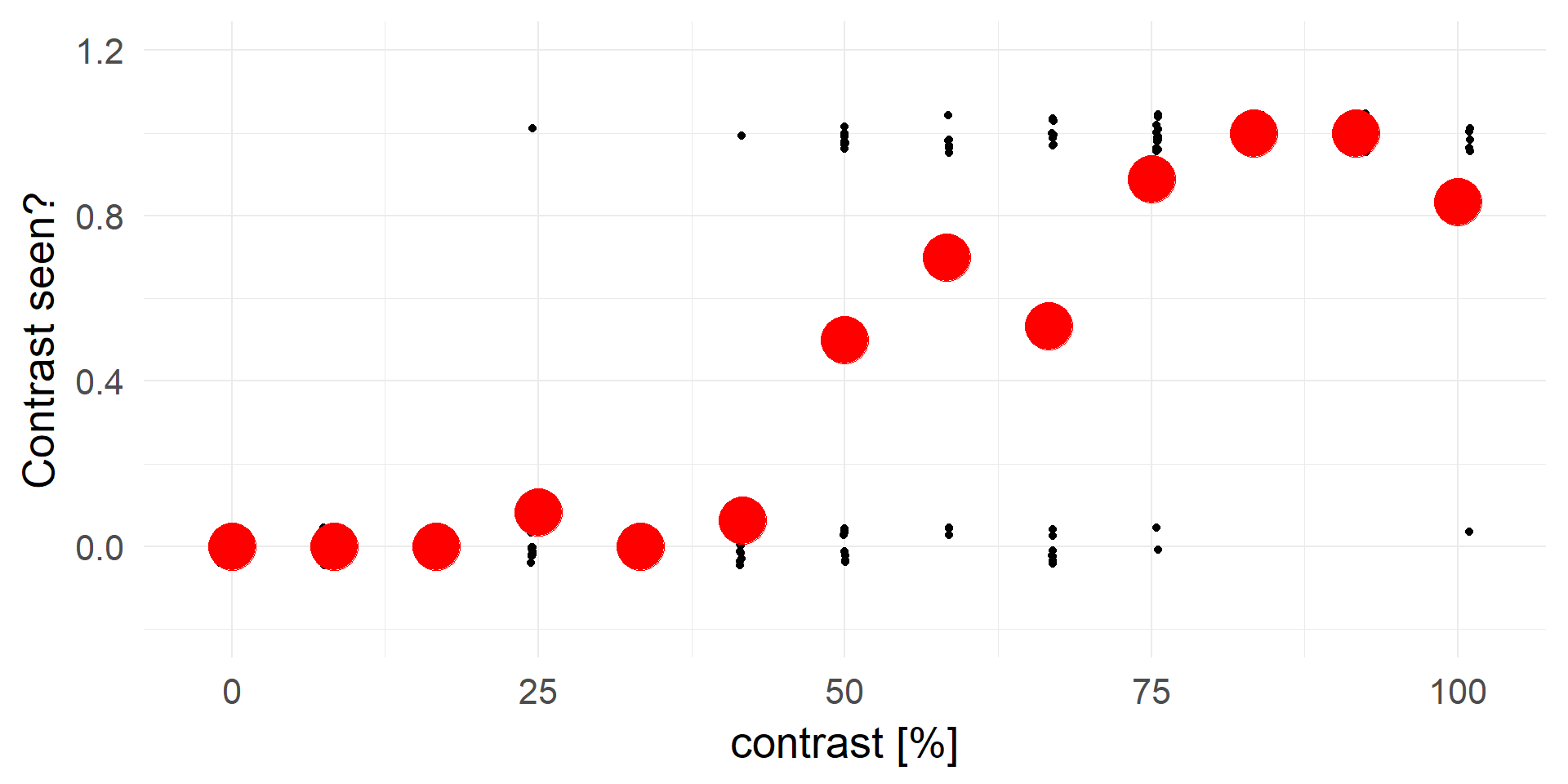## Let’s try linear regression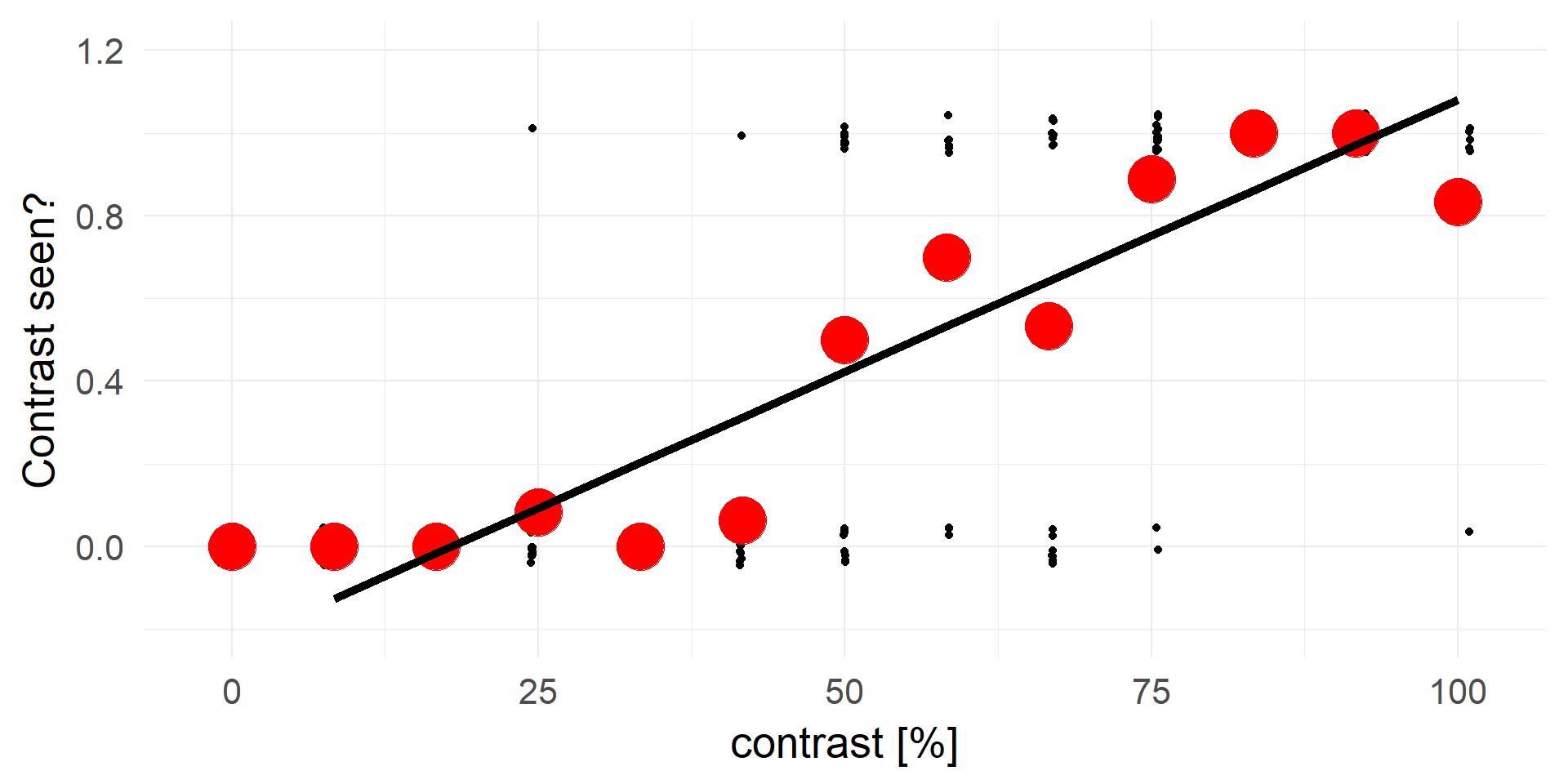There are no negative probabilities. What to do?

## Truncating everything smaller 0 / larger 1: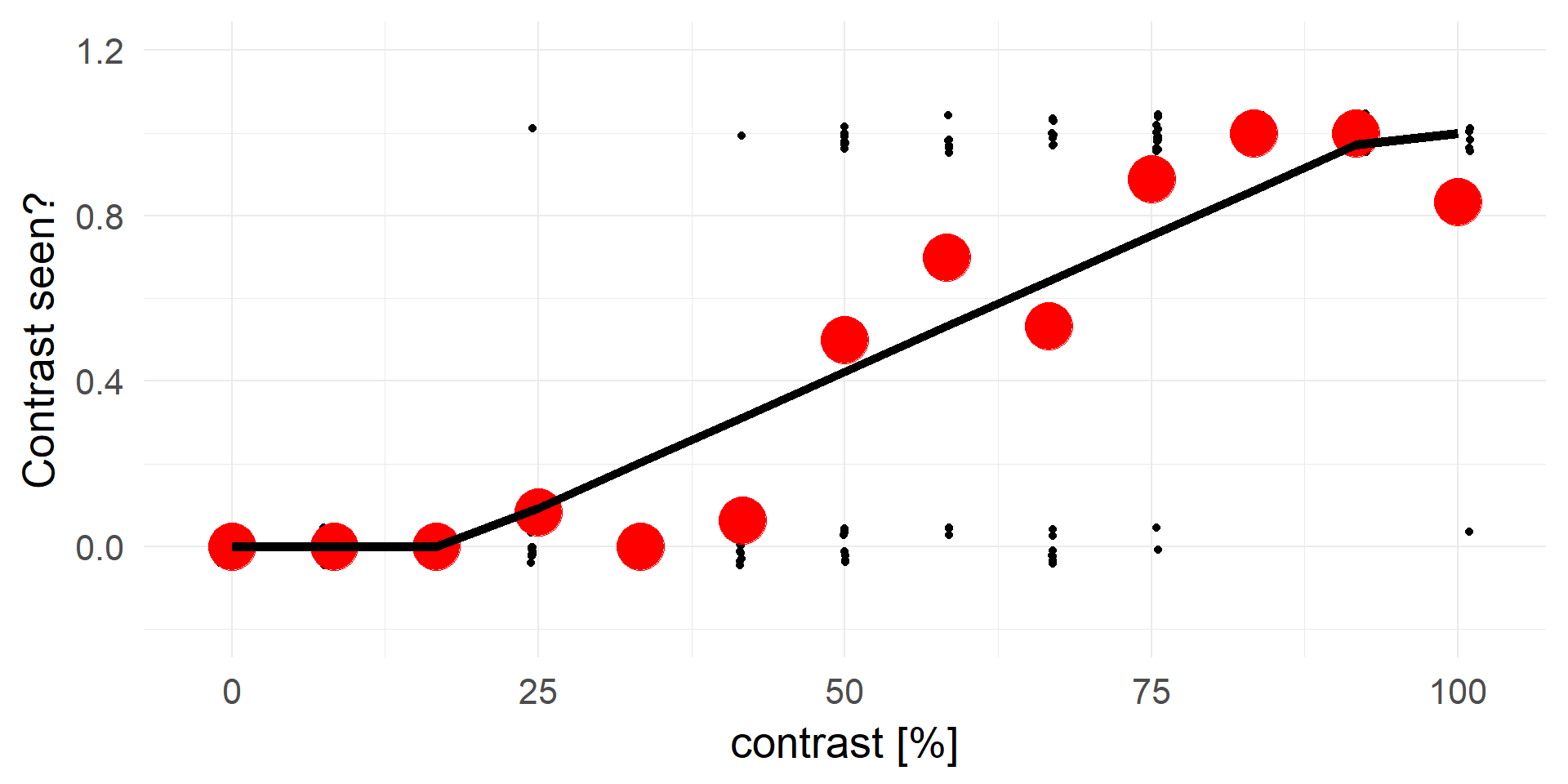A contrast of ~16% will be rejected in 100% of cases.

A strong statement!

## A better fit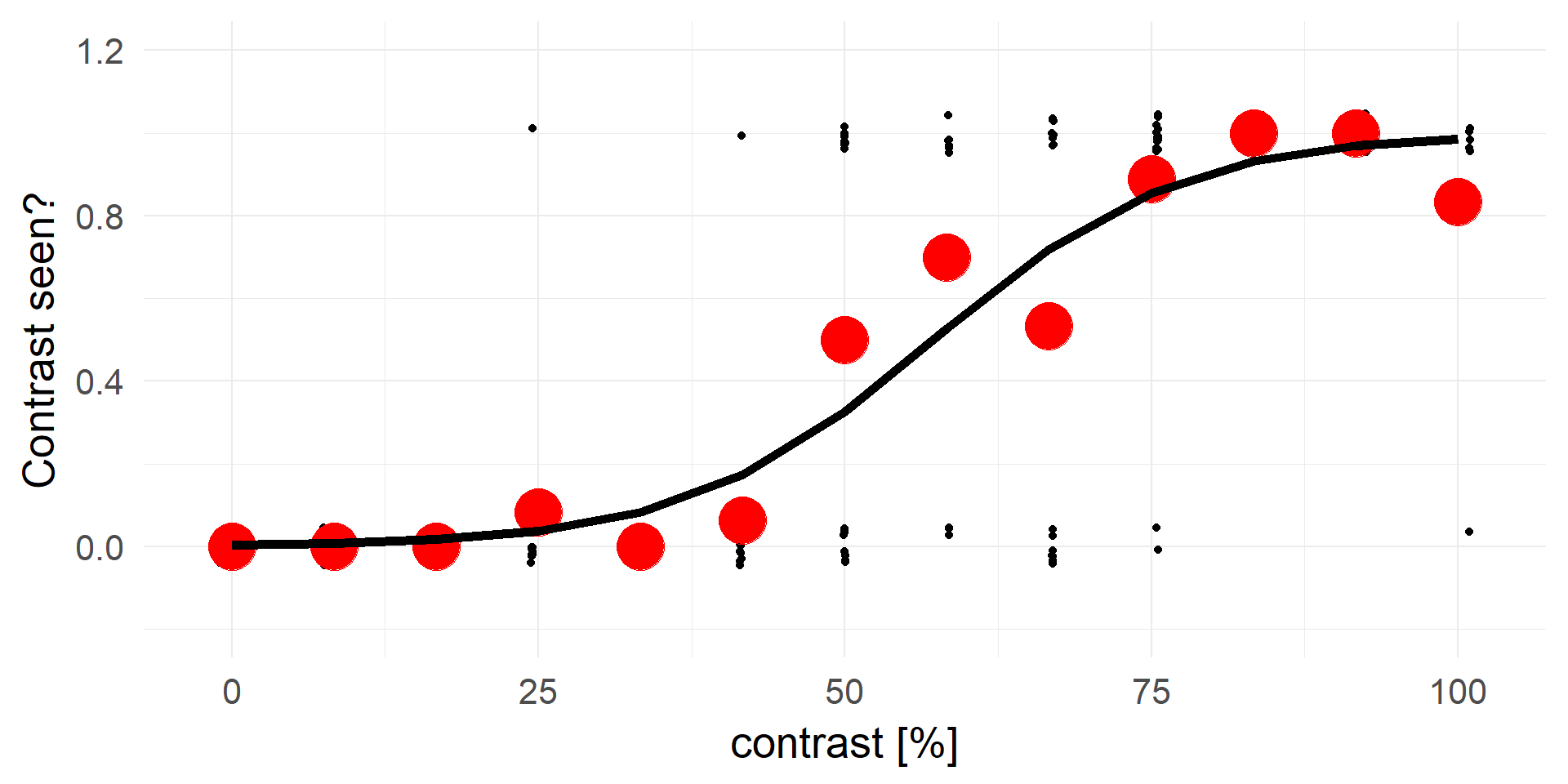## transforming y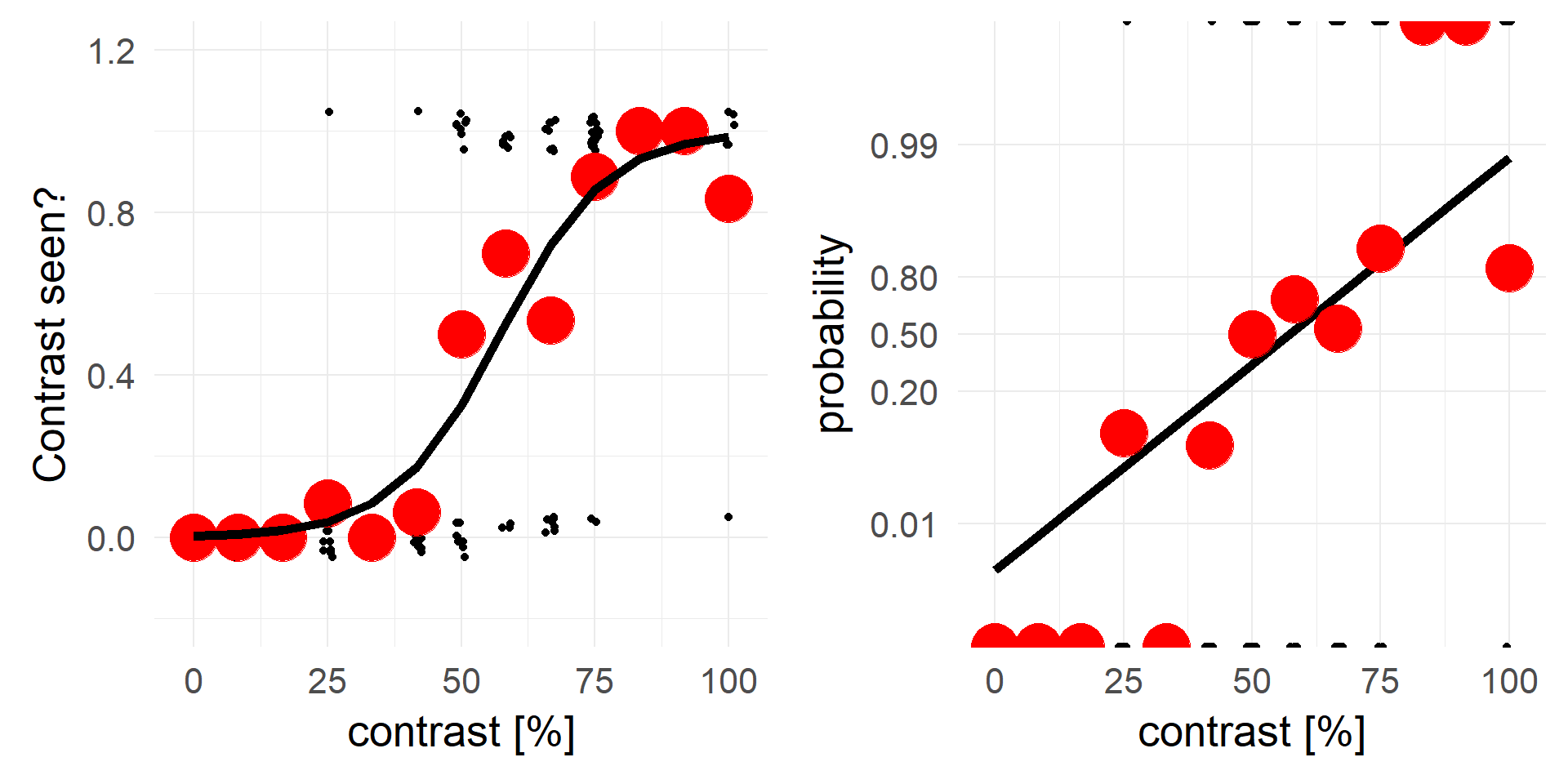An alternative view, same data but different y-axis

## logit & inv.logit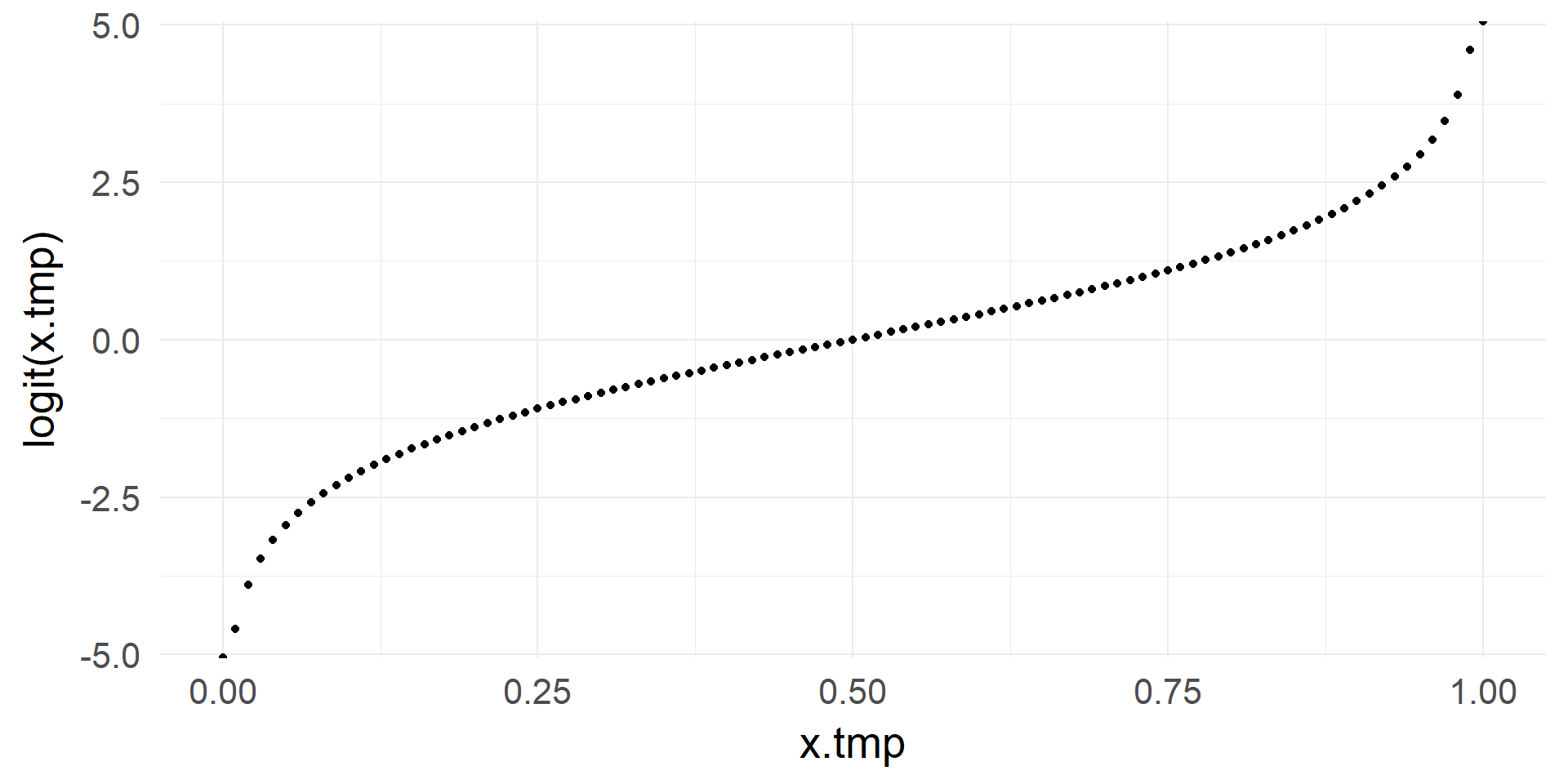From $$[0, 1]$$ to $$[-\infty, +\infty]$$

$x = log(\frac{p}{1-p})$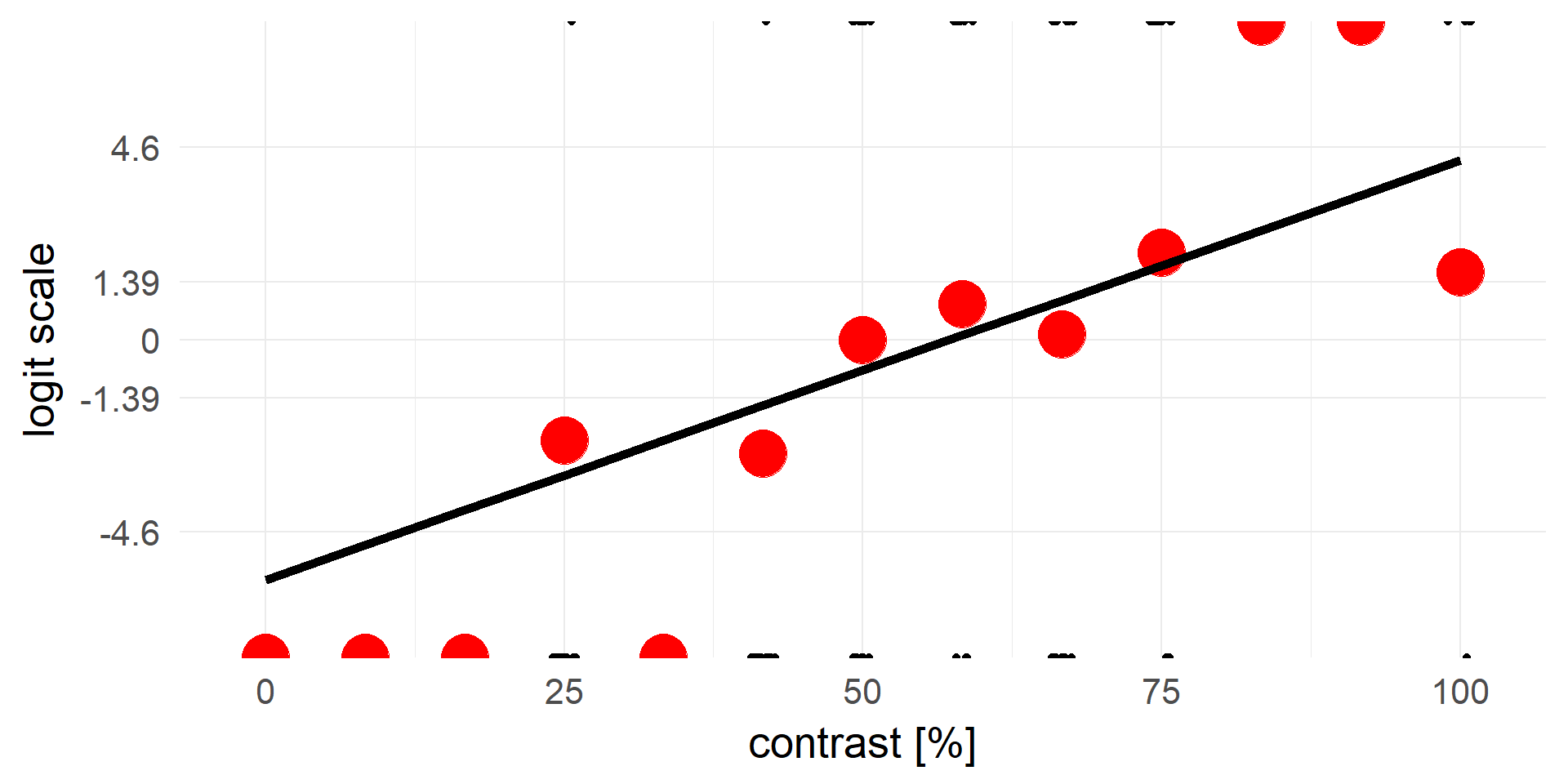The domain we are working in goes from $$-\infty$$ to $$+\infty$$. We assume our relationships are additive in this domain

# Overview

• Binary Outcomes
• Example
• Logistic regression
• Units, odds, relative risks
• Interpretation of coefficients
• Checking the model
• Inference
• Poisson Regression
• GLMs
• Assumptions

## logistic regression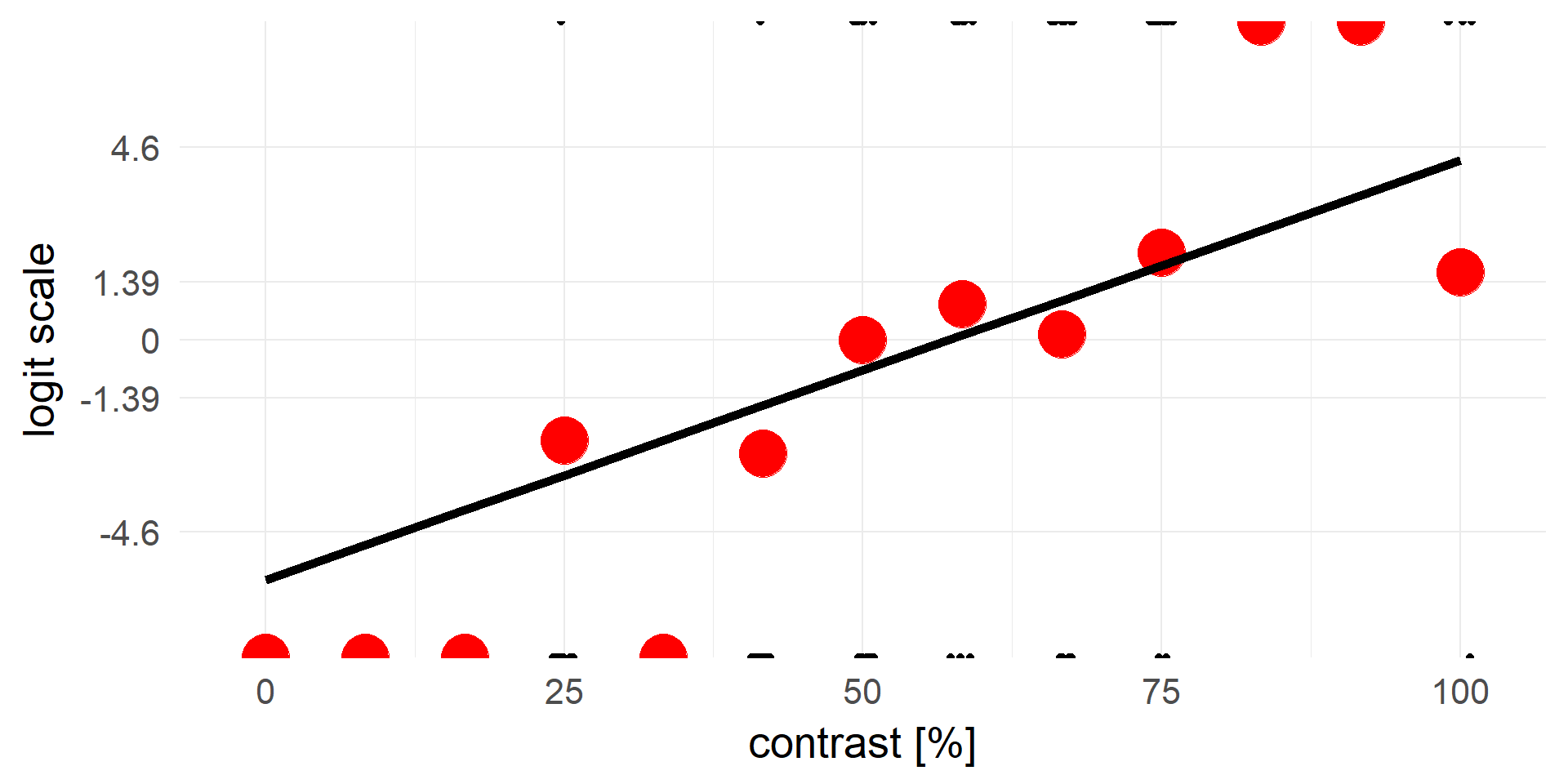$$y = invlogit(X\beta + e)$$ <=> $$logit(y) = X\beta + e$$

$y = g^-1( X\beta + e)$

yes! that is the g from GLM

We call this logistic regression

## Running a logistic regression in R

res = glm(formula = response ~ 1 + contrast, data=d,family=binomial)
summary(res)
##
## Call:
## glm(formula = response ~ 1 + contrast, family = binomial, data = d)
##
## Deviance Residuals:
##     Min       1Q   Median       3Q      Max
## -2.9298  -0.4171  -0.1217   0.5597   2.5579
##
## Coefficients:
##             Estimate Std. Error z value Pr(>|z|)
## (Intercept) -5.73617    0.91880  -6.243 4.29e-10 ***
## contrast     0.10014    0.01556   6.437 1.22e-10 ***
## ---
## Signif. codes:  0 '***' 0.001 '**' 0.01 '*' 0.05 '.' 0.1 ' ' 1
##
## (Dispersion parameter for binomial family taken to be 1)
##
##     Null deviance: 205.78  on 149  degrees of freedom
## Residual deviance: 103.39  on 148  degrees of freedom
## AIC: 107.39
##
## Number of Fisher Scoring iterations: 6

## the interplay of our two predictors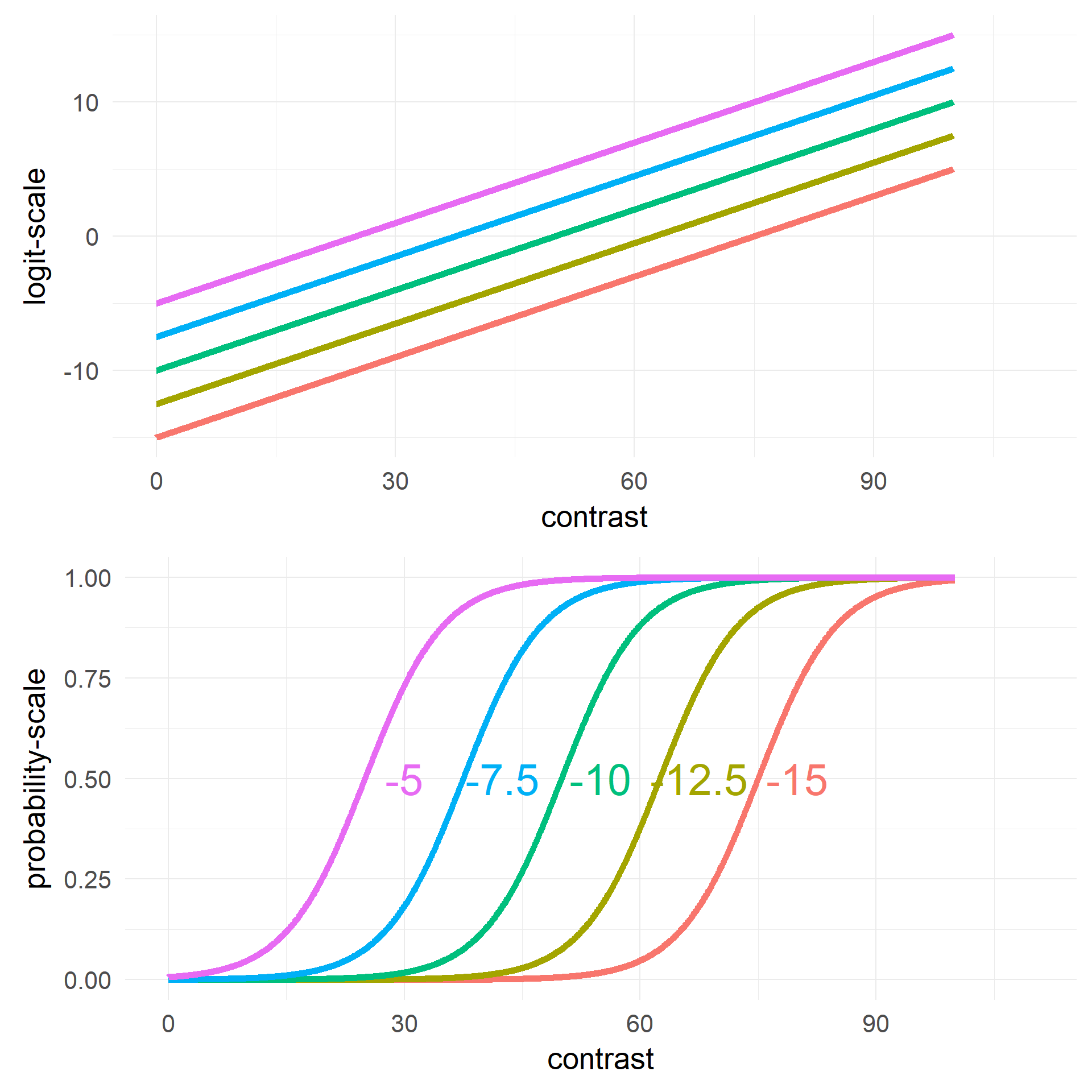Changing Intercept $$\beta_0$$

$y = g^-1(\beta_0 + X_1\beta_1 + e)$

# Overview

• Binary Outcomes
• Example
• Logistic regression
• Units, odds, relative risks
• Interpretation of coefficients
• Checking the model
• Inference
• Poisson Regression
• GLMs
• Assumptions

## What are the units on the y-axis / the coefficients?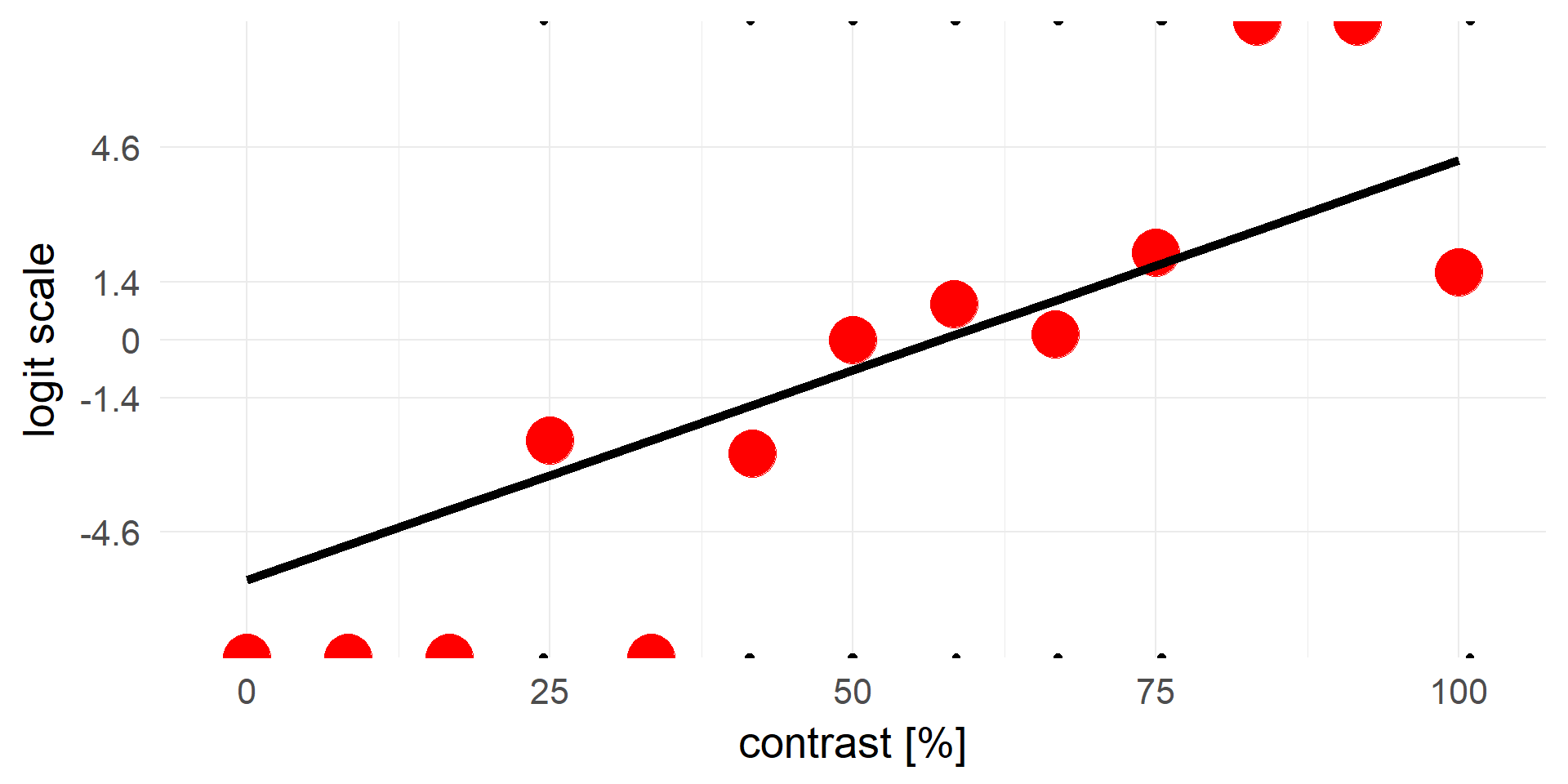$invlogit = \frac 1 {1+e^{-x}}$

$logit = log(\frac p {1-p}) = log(odds)$

## What are odds?

Odds are a somewhat unintuitive quantity: $p(coxi==nerd) = 0.75$

$odds(coxi==nerd) = \frac{p(coxi==nerd)}{p(coxi \neq nerd)} = \frac{0.75}{1-0.75} = \frac{0.75}{0.25} = 3$

In words: the odds for an cogsci to be a nerd are 3 to 1

For 3 nerd coxis you will find 1 non-nerd

## A closer look at the logit function

$odds = \frac{p}{1-p}$ ($$odds \in [0,+\infty]$$, halfway there)

taking the log()

$log(odds) = logit = log(\frac{p}{1-p})$

This is a mapping from $$p \in [0,1]$$ to $$logit(p) \in [-\infty +\infty]$$

## Background: Differences in probabilities, relative risk vs risk difference

• $$p(coxi==nerd) = 0.75$$

• $$p(psycho==nerd) = 0.6$$

• Risk Difference: 0.75 - 0.6 => coxis are 15 percentage points more likely to be nerds
• Relative Risk: $$\frac{0.75}{0.6} = 1.25$$ => Coxis are 25% more likely to be nerds

• Relative Risks are intuitive but non symmetric:
• $$\frac{p(X)}{p(Y)} = \frac{0.99}{0.98} = 1.01$$ => X is 1% more likely than Y
• $$\frac{p(\neg X)}{p(\neg Y)} = \frac{0.01}{0.02} = 0.5$$ => not X is 50% more likely than not Y

## The problem with relative risks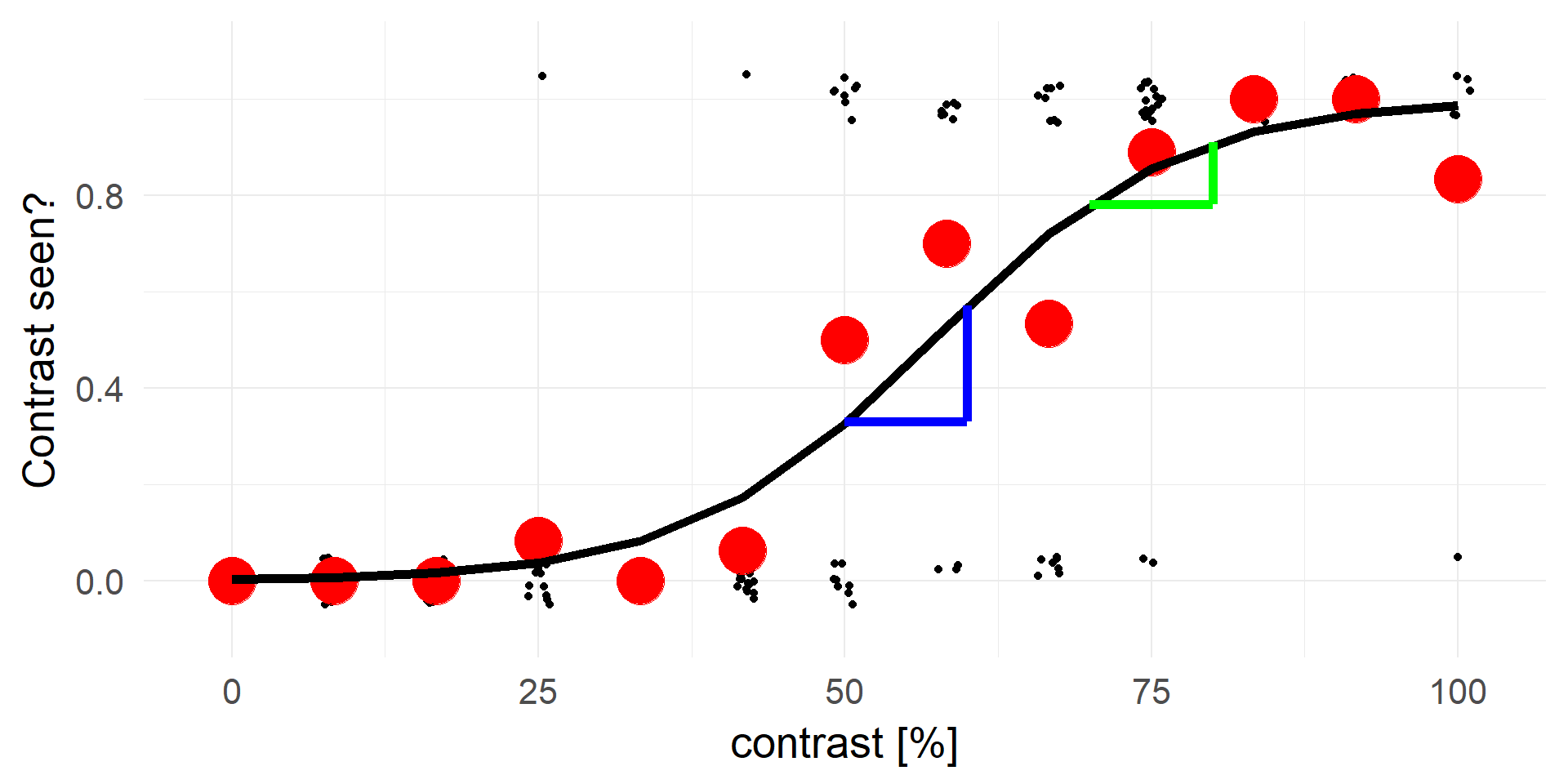p(X|contrast=60) / p(X|contrast=50) = 0.57/0.33 = 1.73

p(X|contrast=80) / p(X|contrast=70) = 0.91/0.78 = 1.17

An contrast increase of 10 on X is not a constant increase in the relative risks.

# Overview

• Binary Outcomes
• Example
• Logistic regression
• Units, odds, relative risks
• Interpretation of coefficients
• Checking the model
• Inference
• Poisson Regression
• GLMs
• Assumptions

## The constant / Intercept

TLDR; $$\beta_0$$ = log-odds if everything else is 0.
• $$\beta_0$$<0: $$p = invlogit(-) < 0.5$$
• $$\beta_0$$==0: $$p = invlogit(0) = 0.5$$
• $$\beta_0$$>0: $$p = invlogit(+) > 0.5$$

• $$y(x) = invlogit(\beta_0 + \beta_1 x)$$
• $$y(x) = \frac{1}{1 + e^{-(\beta_0 + \beta_1 x)}}$$
• $$y(x=0) = \frac{1}{1 + e^{-\beta_0}}$$

## In our example

coef(glm(response~contrast,data=d,family=binomial)) # log-odds
## (Intercept)
##   -5.736166
invlogit(coef(glm(response~contrast,data=d,family=binomial))) # probability
## (Intercept)
## 0.003216738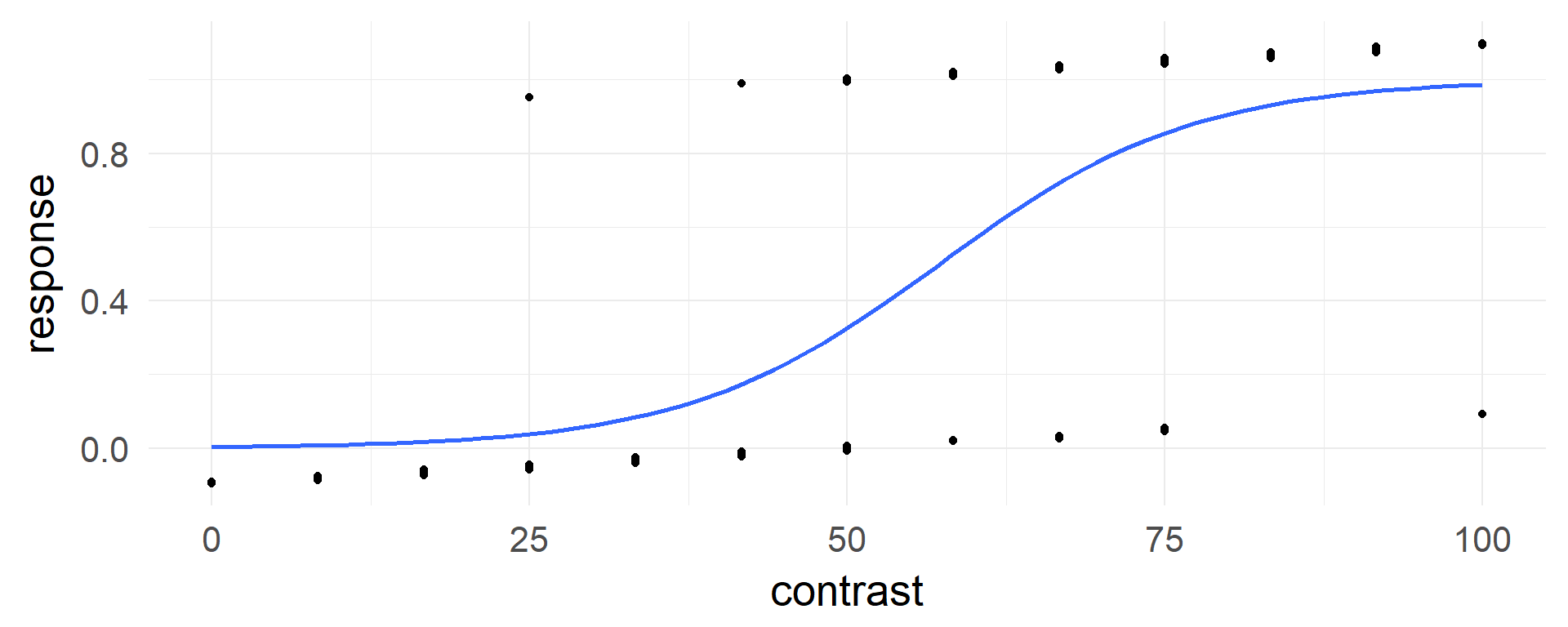## Interpreting the slope

TLDR; $$\beta_1$$ = how much a change of x+1 increases the log(odds-ratio)

$log(odds) = \color{red}{logit(\hat y(x))} = \beta_0 + \beta_1 x$
An increase by $$1*\beta_1$$ : $$\color{green}{logit(\hat y(x+1))} = \beta_0 + \beta_1 (x+1)$$

Taking the difference:

$\color{green}{logit(\hat y(x+1))} - \color{red}{logit(\hat y(x))} = \color{green}{\beta_0 + \beta_1 (x+1)} - (\color{red}{\beta_0 + \beta_1 x} )= \beta_1$
Using the log-rule on the left side ($$log(odds_a) - log(odds_b) = log(\frac {odds_a} {odds_b} )$$)

$\beta_1 = \color{green}{logit(\hat y(x+1))} - \color{red}{logit(\hat y(x))} = \color{green}{log(odds(x+1)} - \color{red}{log(odds(x))}= log(\color{blue}{\frac{odds(x+1)}{odds(x)}})$
$\beta_1 = log(\color{blue}{odds-ratio})$

## What are odds-ratios?

$odds(coxi==nerd) = 3$

$odds(psycho==nerd) = 1.5$

$odds-ratio = \frac{3}{1.5} = 2$

The odds that coxis are nerds are 2x as high than the odds that psychology students are nerds.

(remember relative risk: Coxis are 1.25 as likely than psychology students to be nerds)

$e^{\beta_1} = odds-ratio( y(x)/y(x+1))$

The odds that you will succeed are $$e^{beta}$$ higher at $$y(x+1)$$ than $$y(x)$$

## Back to the example

co = coef(glm(response~contrast,data=d,family=binomial)) # log-odds ratio
co["contrast"]
##  contrast
## 0.1001421
exp(co["contrast"]) # odds ratio
## contrast
## 1.105328

With a step of 1, the odds change by ~1.1

With a step of 10, the odds change by ~2.7

## Coming back to relative risks

Interpretation of odds ratios is difficult and unintuitive. I always try to give examples, i.e. what is the relative risks between two outcomes.

What is the relative risk between contrast = 50 and contrast = 80?

a = invlogit(co["(Intercept)"]+co["contrast"] * 80)
b = invlogit(co["(Intercept)"]+co["contrast"] * 50)

we have ~280% more successes at contrast = 80 than at contrast = 50

# Intermediate Summary

$y = g^-1(\beta X + e)$

$$y = invlogit(\beta X + e)$$ <=> $$logit(y) = \beta X + e$$

• Binary data (0/1, True/False)
• Units are log(odds)
• one of many link-functions of GLMs
• It is possible to use other functions that map $$p \in [0,1]$$ to $$logit(p) \in [-\infty +\infty]$$, e.g. probit (cumulative normal). But the units are not as easily interpretable as odds (!)

# Overview

• Binary Outcomes
• Example
• Logistic regression
• Units, odds, relative risks
• Interpretation of coefficients
• Checking the model
• Inference
• Poisson Regression
• GLMs
• Assumptions

## Residuals

res = glm(formula = response ~ 1 + contrast, data=d,family=binomial)
summary(res)
##
## Call:
## glm(formula = response ~ 1 + contrast, family = binomial, data = d)
##
## Deviance Residuals:
##     Min       1Q   Median       3Q      Max
## -2.9298  -0.4171  -0.1217   0.5597   2.5579
##
## Coefficients:
##             Estimate Std. Error z value Pr(>|z|)
## (Intercept) -5.73617    0.91880  -6.243 4.29e-10 ***
## contrast     0.10014    0.01556   6.437 1.22e-10 ***
## ---
## Signif. codes:  0 '***' 0.001 '**' 0.01 '*' 0.05 '.' 0.1 ' ' 1
##
## (Dispersion parameter for binomial family taken to be 1)
##
##     Null deviance: 205.78  on 149  degrees of freedom
## Residual deviance: 103.39  on 148  degrees of freedom
## AIC: 107.39
##
## Number of Fisher Scoring iterations: 6

## Deviance / Residuals

$$\theta_i = \hat{y}_i = invlogit(\beta X)$$

• $$LL(y|\hat y) =$$ loglikelihood $$=log(\prod{\hat{y_i}^{y_i} (1-\hat{y_i})^{(1-y_i)}})$$
• for a single data point simplifies to
• if $$y_i = 1$$:    $$log(\hat{y_i})$$      if $$y_i = 0$$:   $$log(1-\hat{y_i})$$
• Deviance Residuals: $$d_i = s_i\sqrt{-2LL(y_i|\hat y_i)}$$ $$s_i = 1$$ if $$y_i = 1$$, $$s_i = -1$$ if $$y_1 = 0$$
• Intuition words: deviance is a measure of how well the model fits a data point.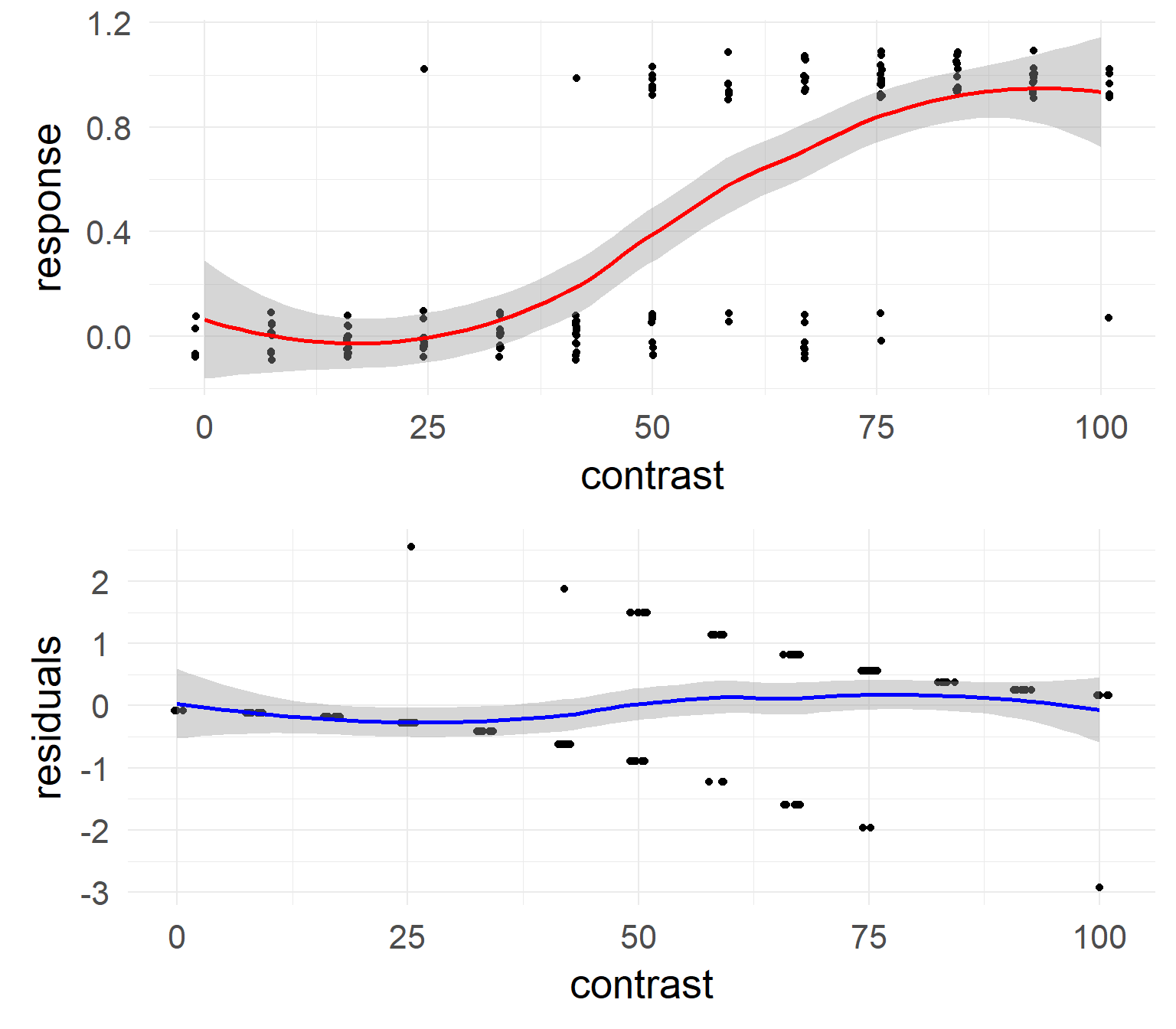## Null & Residual Deviance

res = glm(formula = response ~ 1 + contrast, data=d,family=binomial)
summary(res)
##
## Call:
## glm(formula = response ~ 1 + contrast, family = binomial, data = d)
##
## Deviance Residuals:
##     Min       1Q   Median       3Q      Max
## -2.9298  -0.4171  -0.1217   0.5597   2.5579
##
## Coefficients:
##             Estimate Std. Error z value Pr(>|z|)
## (Intercept) -5.73617    0.91880  -6.243 4.29e-10 ***
## contrast     0.10014    0.01556   6.437 1.22e-10 ***
## ---
## Signif. codes:  0 '***' 0.001 '**' 0.01 '*' 0.05 '.' 0.1 ' ' 1
##
## (Dispersion parameter for binomial family taken to be 1)
##
##     Null deviance: 205.78  on 149  degrees of freedom
## Residual deviance: 103.39  on 148  degrees of freedom
## AIC: 107.39
##
## Number of Fisher Scoring iterations: 6

Null Deviance$$^2$$: $$2(LL(M_{saturated}) - LL(M_0))$$

Residual Deviance$$^2$$: $$2(LL(M_{saturated}) - LL(M_1))$$

# Overview

• Binary Outcomes
• Example
• Logistic regression
• Units, odds, relative risks
• Interpretation of coefficients
• Checking the model
• Inference
• Poisson Regression
• GLMs
• Assumptions

## Model comparison

m0 = glm(response~1           ,data=d,family=binomial)
m1 = glm(response~1 + contrast,data=d,family=binomial)
anova(m0,m1,test='LRT')
## Analysis of Deviance Table
##
## Model 1: response ~ 1
## Model 2: response ~ 1 + contrast
##   Resid. Df Resid. Dev Df Deviance  Pr(>Chi)
## 1       149     205.78
## 2       148     103.39  1   102.39 < 2.2e-16 ***
## ---
## Signif. codes:  0 '***' 0.001 '**' 0.01 '*' 0.05 '.' 0.1 ' ' 1

The predictor contrast is highly significant

Which is (in the case of one predictor) exactly the same as:

Null Deviance - Residual Deviance: $$(LL(M_{1}) - LL(M_{0}))$$

# Overview

• Binary Outcomes
• Example
• Logistic regression
• Units, odds, relative risks
• Interpretation of coefficients
• Checking the model
• Inference
• Poisson Regression
• GLMs
• Assumptions

## Poisson Regression

Count-data has an average rate per time unit: $$\lambda$$

• Matings of animals per year (Biology)
• How many/much seizures/headaches/pain per week (Medicine)
• How many spikes per 10ms bin (Neuroscience)
• How many eve-movements per minute/trial (CogPsy)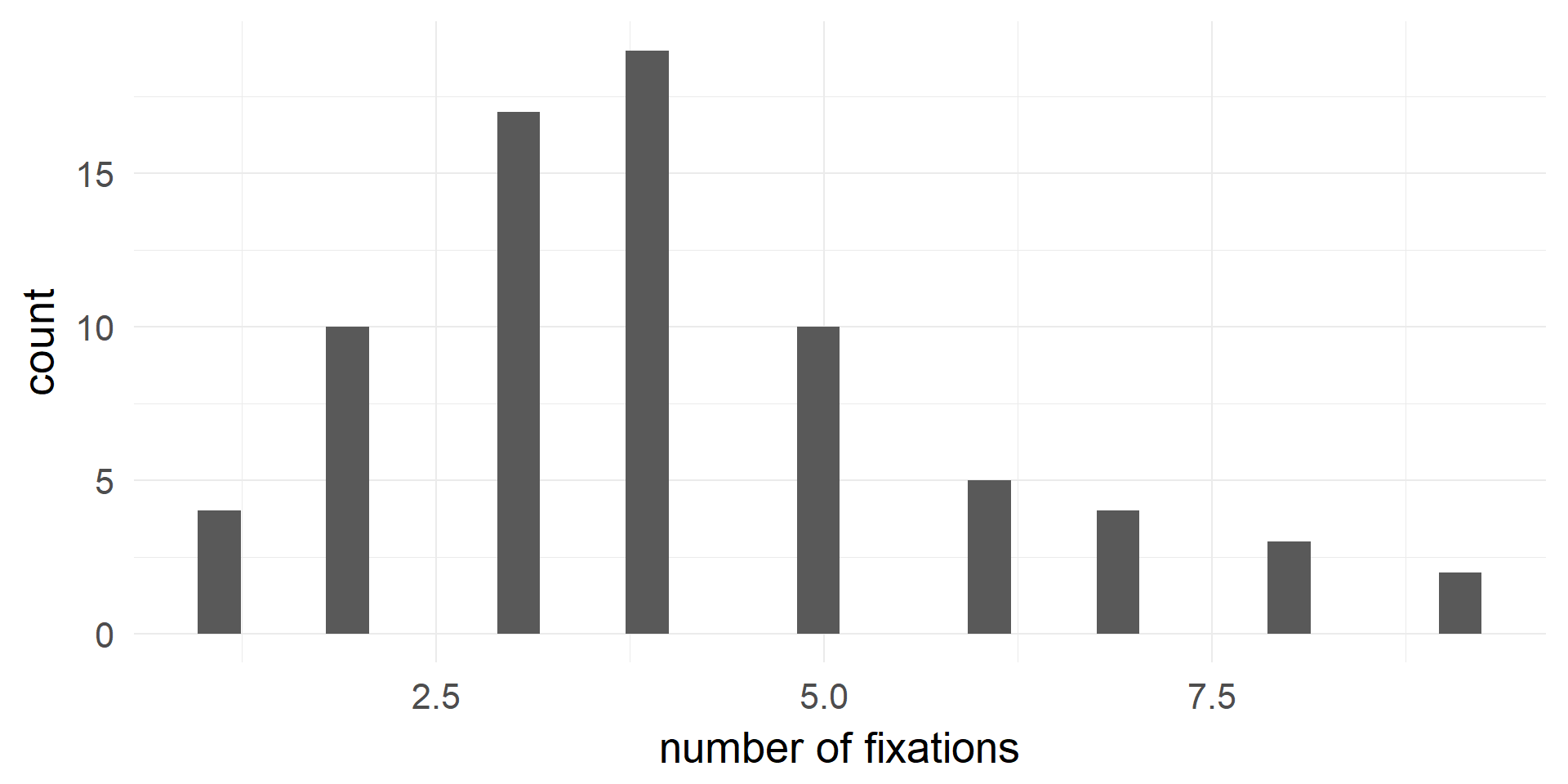## (fictional) Example: Poisson Regression## fitting in R and Interpretation

m1 = glm(data=df.pois,formula = fixations~coffee*tea,family=poisson)
summary(m1)
##
## Call:
## glm(formula = fixations ~ coffee * tea, family = poisson, data = df.pois)
##
## Deviance Residuals:
##      Min        1Q    Median        3Q       Max
## -2.73861  -0.69280   0.06776   0.59125   2.28465
##
## Coefficients:
##             Estimate Std. Error z value Pr(>|z|)
## (Intercept)  1.48160    0.10660  13.899   <2e-16 ***
## coffee       0.09737    0.14722   0.661    0.508
## tea         -0.15985    0.15715  -1.017    0.309
## coffee:tea   0.29467    0.20980   1.405    0.160
## ---
## Signif. codes:  0 '***' 0.001 '**' 0.01 '*' 0.05 '.' 0.1 ' ' 1
##
## (Dispersion parameter for poisson family taken to be 1)
##
##     Null deviance: 75.082  on 79  degrees of freedom
## Residual deviance: 67.630  on 76  degrees of freedom
## AIC: 338.54
##
## Number of Fisher Scoring iterations: 4

link-function g = $$log(\lambda) = X\beta$$

$$\lambda = e^{X\beta }$$

remember $$e^{a+b} = e^ae^b$$ => Poisson parameters are multiplicative!

## Interpretation of coefficients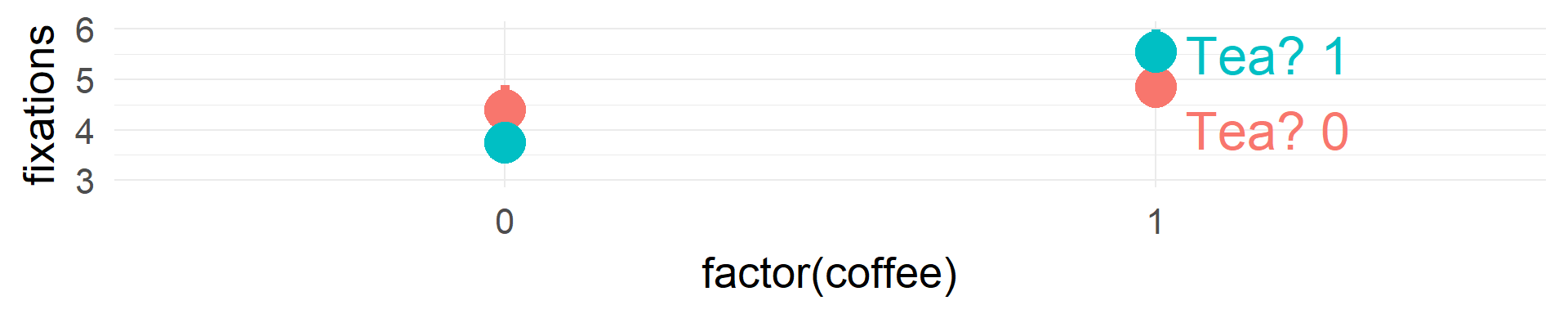(Intercept) coffee tea coffee:tea
coef(m1) 1.48 0.1 -0.16 0.29
exp(coef(m1)) 4.40 1.1 0.85 1.34

Condition $$log(\\lambda) = \\sum coef$$ $$\\lambda = \\prod e^{coef} = e^{\\sum coef}$$ $$\\lambda$$
Tea = 0 & Coffee = 0 1.48 4.4 4.4
Tea = 1 & Coffee = 0 1.48-0.16 4.4*0.85 3.74
Tea = 0 & Coffee = 1 1.48+0.09 4.4*1.1 4.84
Tea = 1 & Coffee = 1 1.48-0.16+0.09+0.29 4.4 * 0.85 * 1.1 5.51
anova(m1,test = 'LRT')
## Analysis of Deviance Table
##
##
## Response: fixations
##
## Terms added sequentially (first to last)
##
##
##            Df Deviance Resid. Df Resid. Dev Pr(>Chi)
## NULL                          79     75.082
## coffee      1   5.4717        78     69.611  0.01933 *
## tea         1   0.0027        77     69.608  0.95859
## coffee:tea  1   1.9782        76     67.630  0.15958
## ---
## Signif. codes:  0 '***' 0.001 '**' 0.01 '*' 0.05 '.' 0.1 ' ' 1

link-function g = $$log(\lambda)$$

# Overview

• Binary Outcomes
• Example
• Logistic regression
• Units, odds, relative risks
• Interpretation of coefficients
• Checking the model
• Inference
• Poisson Regression
• GLMs
• Assumptions

## The GLM family

Probability Distribution Link-Function (g) Statistical name
Normal $$\beta X = y$$ Linear Regression
Bernoulli $$\beta X = log(\frac {p} {1-p})$$ Logistic Regression
Poisson $$\beta X = log(\lambda)$$ Poisson Regression
ExponentialGamma $$\beta X = \frac 1 x$$ Gamma Regression

## Assumptions

• all GLMs data points are independent
• the variance follows the mean in a specific way:
• Binomial: $$\sigma^2 = \mu(1-\mu)$$
• Poisson: $$\sigma^2 = \mu$$
• Gamma: $$\sigma^2 = \mu^2$$
• Normal: $$\sigma^2 = 1$$ (constant)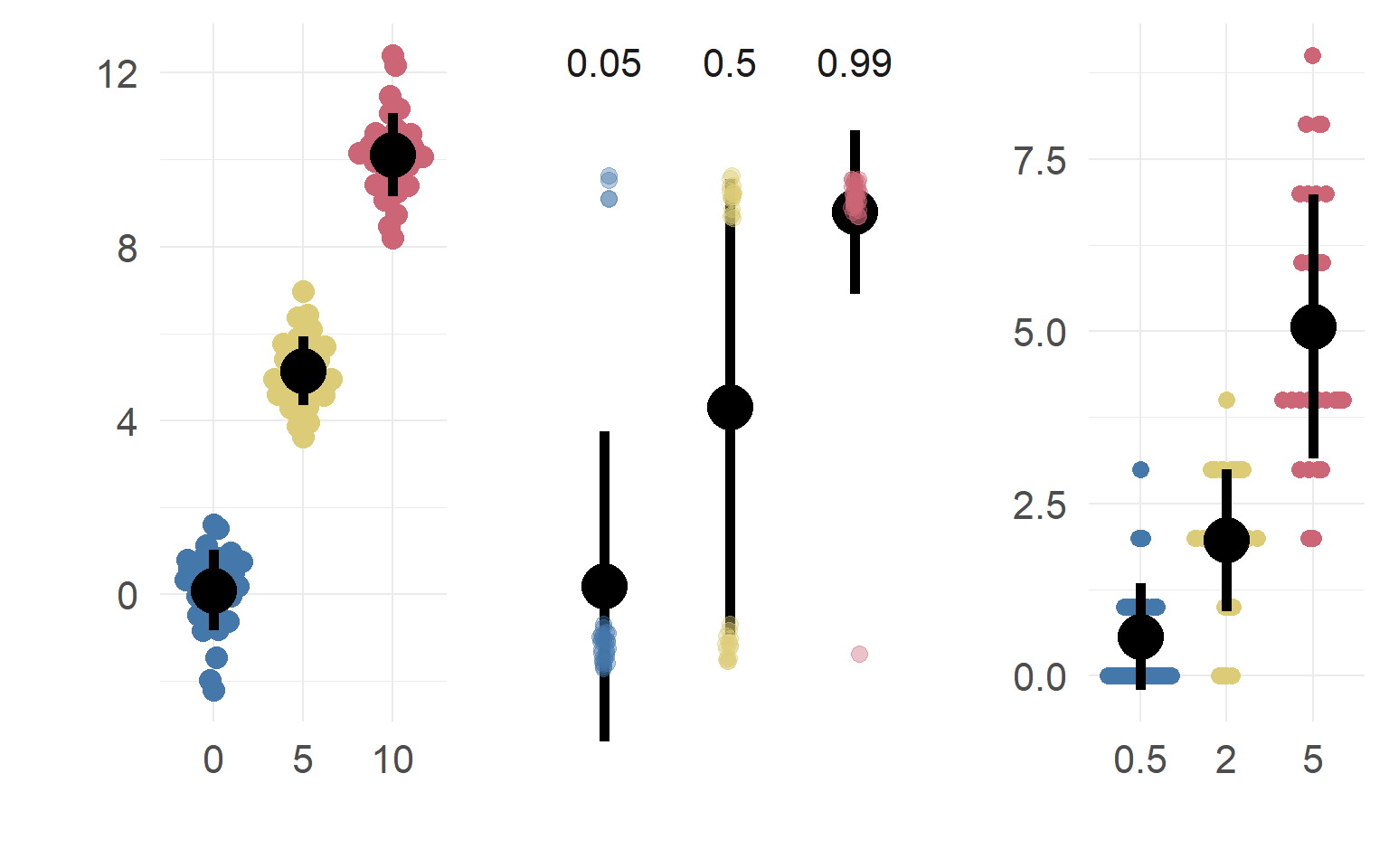## Poisson: A simple example when var != mean

dataSubset = df.pois$coffee==0 & df.pois$tea==0
mean(df.pois$fixations[dataSubset]) ##  4.4 var (df.pois$fixations[dataSubset])
##  4.778947
mean(df.pois$fixations[dataSubset]*2) ##  8.8 var (df.pois$fixations[dataSubset]*2)
##  19.11579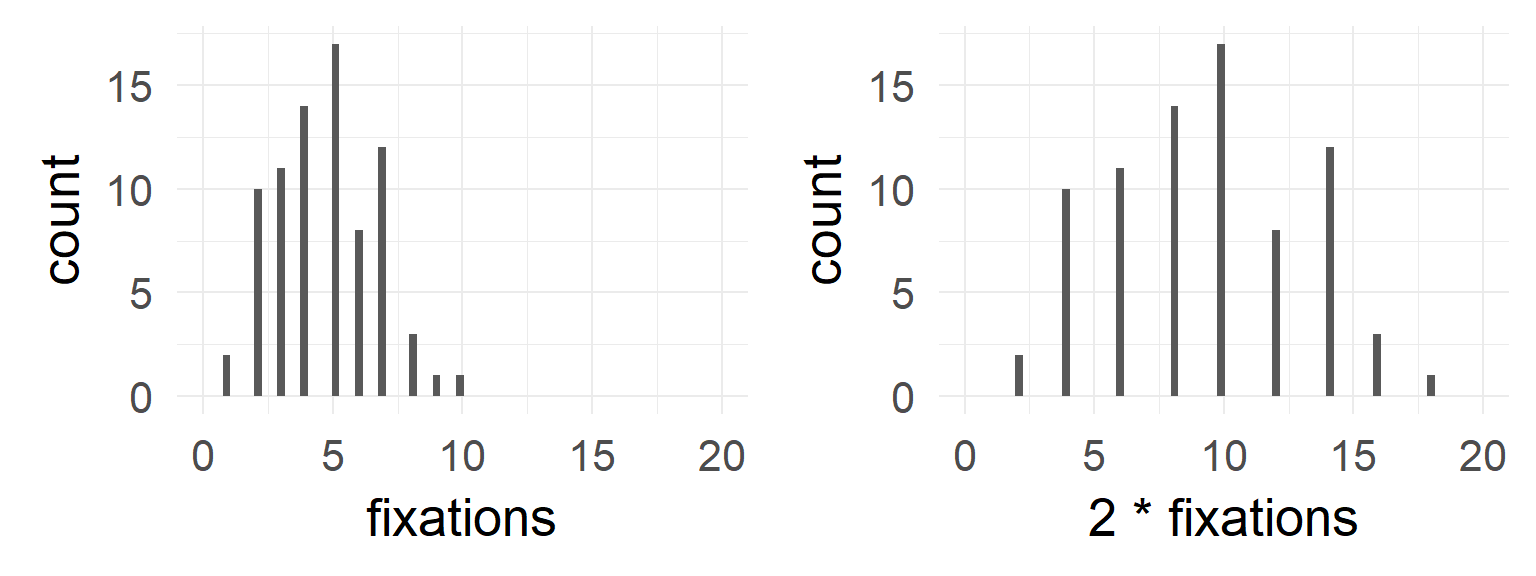## Binomial / Logistic regression

Impossible to have under/overdispersion with only an intercept

$$\sigma^2 = \mu(1-\mu)$$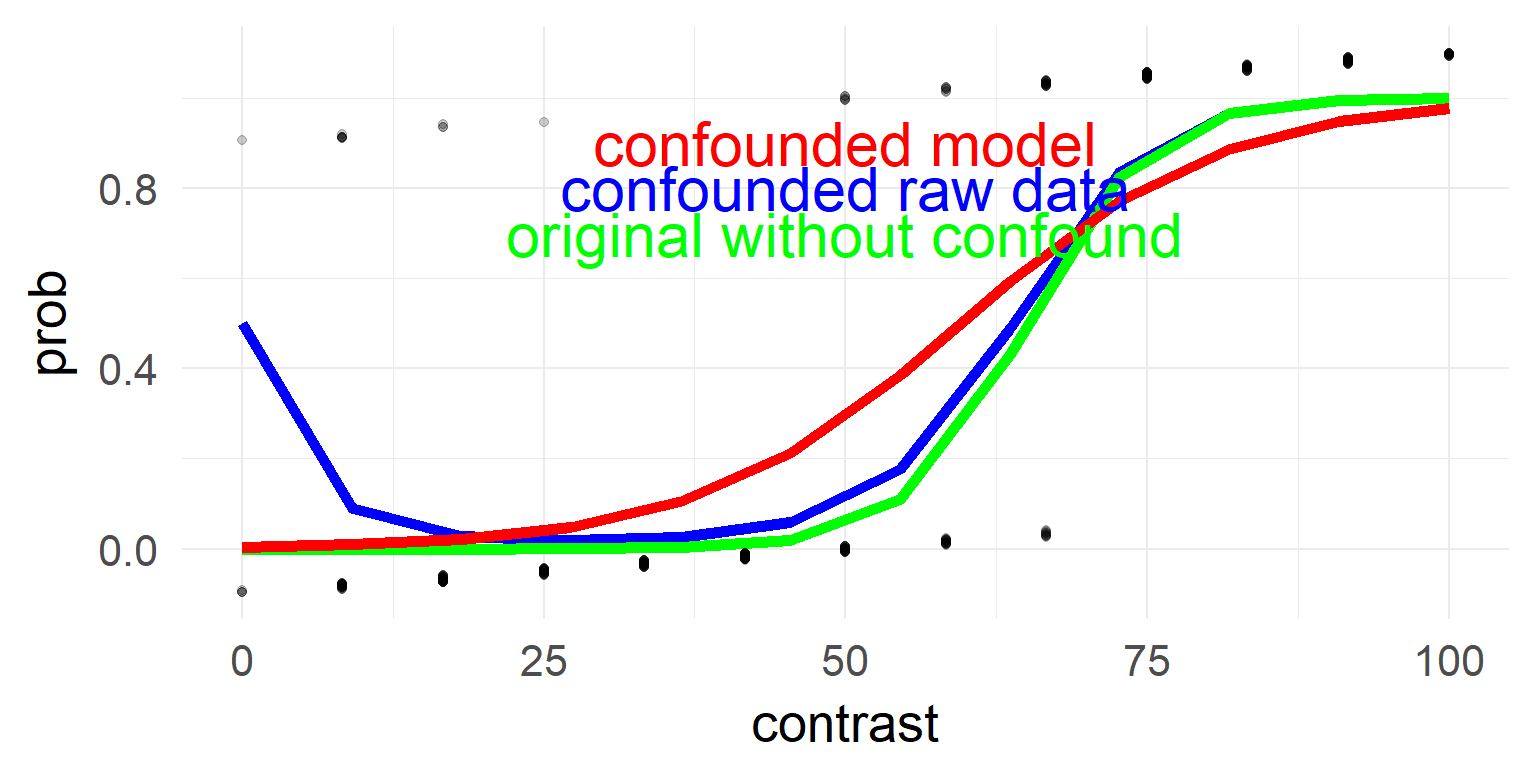# Calculate dispersion parameter
sum(resid(mres, type = "pearson")^2) / df.residual(mres) # should be 1
##  3.384014

## deviance residual plot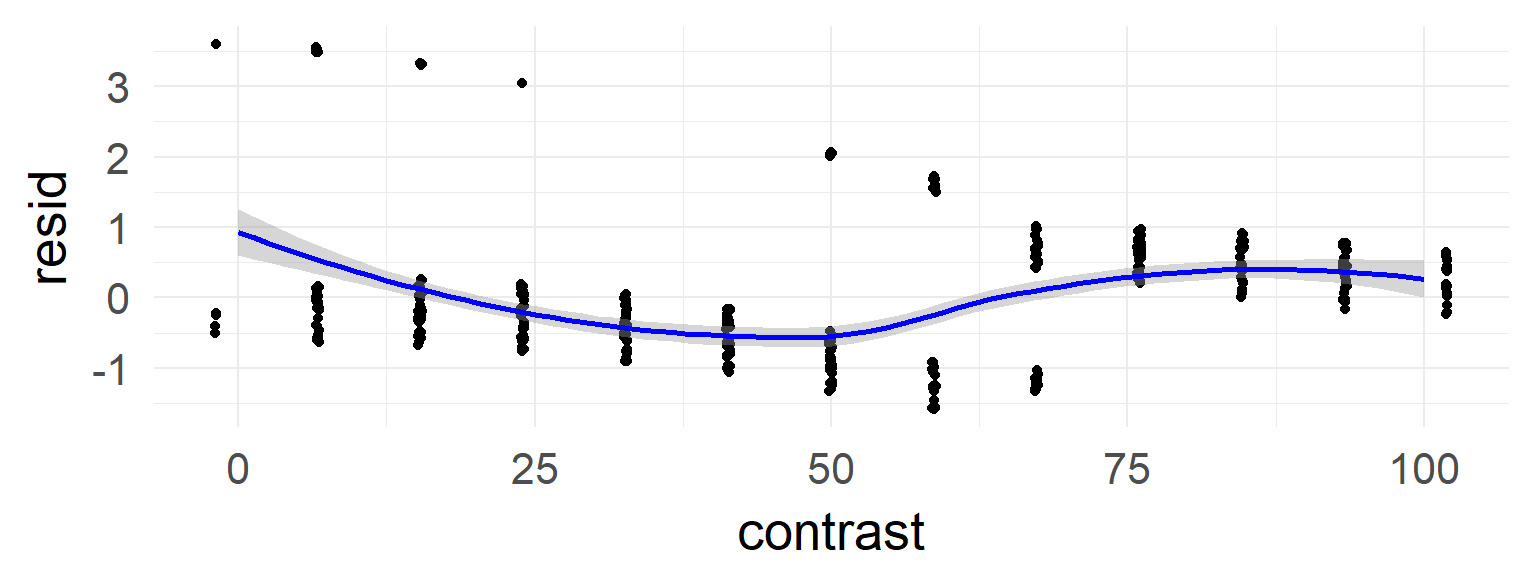In the case of the confound a non-linearity can be seen.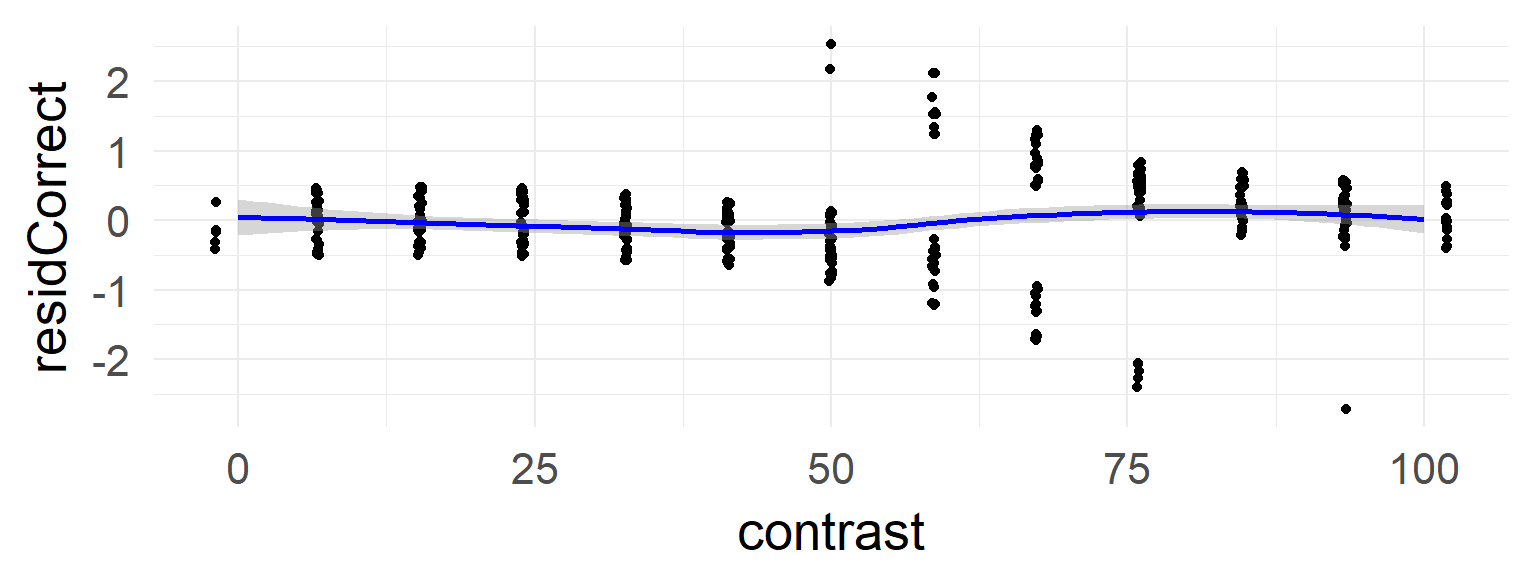This is absent in the data without the confound

## What to do?

Under/Overdispersion can occur in all GLMs (except normal)

### Overdispersion comes mostly from:

• Missing Factors
• Non-linear effects
• outliers

### How to find out?

• Check relation between variance and mean
• logistic: Dispersion parameter read more
• Poisson: mean / variance for binned data

### How to compensate

• Difficult in general
• Quasibinomial / quasipoisson
• Check out DHARMa (read more)

## Excourse: Quasilikelihood example

Estimate an additional variance scaling factor. Thus (e.g. for Poisson):

$Var = \phi \cdot mean$

  mres1 = glm(data=d,response~ 1 + contrast,family=binomial)
##  "             Estimate Std. Error z value Pr(>|z|)    "
##  "(Intercept) -5.514545   0.522216  -10.56   <2e-16 ***"
##  "contrast     0.092564   0.008715   10.62   <2e-16 ***"
##  "(Dispersion parameter for binomial family taken to be 1)"
sum(resid(mres1, type = "pearson")^2) / df.residual(mres1)
##  3.384014
mres2 = glm(data=d,response~ 1 + contrast,family=quasibinomial)
##  "            Estimate Std. Error t value Pr(>|t|)    "
##  "(Intercept) -5.51455    0.96065  -5.740 1.88e-08 ***"
##  "contrast     0.09256    0.01603   5.774 1.56e-08 ***"
##  "(Dispersion parameter for quasibinomial family taken to be 3.384015)"

Quasi-likelihoods make model comparisons more difficult